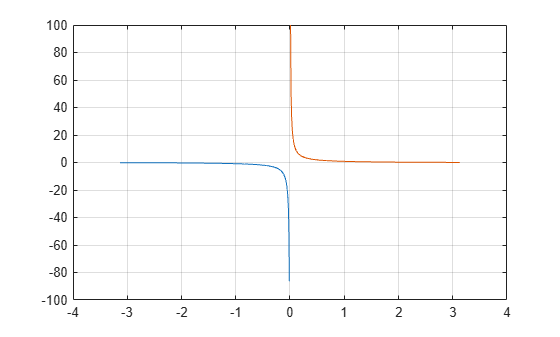# csch

Hyperbolic cosecant

## Syntax

``Y = csch(X)``

## Description

example

````Y = csch(X)` returns the hyperbolic cosecant of the elements of `X`. The `csch` function operates element-wise on arrays. The function accepts both real and complex inputs. All angles are in radians.```

## Examples

collapse all

Create a vector and calculate the hyperbolic cosecant of each value.

```X = [0 pi 2*pi 3*pi]; Y = csch(X)```
```Y = 1×4 Inf 0.0866 0.0037 0.0002 ```

Plot the hyperbolic cosecant over the domain $-\pi and $0

```x1 = -pi+0.01:0.01:-0.01; x2 = 0.01:0.01:pi-0.01; y1 = csch(x1); y2 = csch(x2); plot(x1,y1,x2,y2) grid on```## Input Arguments

collapse all

Input angles in radians, specified as a scalar, vector, matrix, multidimensional array, table, or timetable.

Data Types: `single` | `double` | `table` | `timetable`
Complex Number Support: Yes

collapse all

### Hyperbolic Cosecant

The hyperbolic cosecant of x is equal to the inverse of the hyperbolic sine

`$\text{csch}\left(x\right)=\frac{1}{\mathrm{sinh}\left(x\right)}=\frac{2}{{e}^{x}-{e}^{-x}}.$`

In terms of the traditional cosecant function with a complex argument, the identity is

`$\text{csch}\left(x\right)=i\mathrm{csc}\left(ix\right)\text{\hspace{0.17em}}.$`

## Version History

Introduced before R2006a

expand all